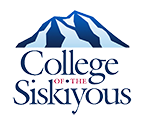# Courses

## MATH 0850 - Elementary Algebra Review

Prerequisite: Qualification by assessment

This course is designed for students needing an intensive and fast-paced extensive review of Algebra and who have previously successfully completed Algebra in high school. Topics include properties of and operations on real numbers, linear equations, linear systems, exponents, polynomials, factoring, rational expressions, radicals, quadratic equations, and parabolas. (NDA)

## MATH 0980 - Intermediate Algebra

Prerequisite: MATH 0850, MATH 0852 or qualification by assessment

This course is a study of algebra including real numbers, linear equations and inequalities, exponents, factoring, rational expressions, radicals, quadratic equations and functions. Emphasis is placed on manipulating literal equations, absolute value equations and inequalities, complex numbers, and systems of equations. (AA).

## MATH 1010 - Finite Mathematics

Prerequisite: MATH 0980 or qualification by assessment

A college-level math course recommended for students majoring in math, biological sciences, or social sciences and required for some business majors. Topics included are linear, exponential functions, systems of linear equations and inequalities, matrix algebra , inverse matrices, linear programming, set theory, DeMorgan's Laws and Venn diagrams, probability and combinatorics, conditional probability, Markov chains and applications to problems in finance including simple and compound interest, future and present value, annuities, sinking funds and amortization. (AA, CSU, UC)

## MATH 1050 - Elementary Statistics

Prerequisite: MATH 0980 or qualification by assessment

The use of probability techniques, hypothesis testing, and predictive techniques to facilitate decision-making. Topics include descriptive statistics; probability and sampling distributions; statistical inference; correlation and linear regression; analysis of variance, chi-square and t-tests; and application of technology for statistical analysis including the interpretation of the relevance of the statistical findings. Applications using data from disciplines including business, social sciences, psychology, life science, health science, and education. (AA, CSU, UC) (C-ID: MATH 110)

## MATH 1100 - College Algebra

Prerequisite: MATH 0980 or qualification by assessment

College level course in algebra for majors in science, technology, engineering, mathematics, and liberal arts: polynomial, rational, radical, exponential, absolute value, and logarithmic functions; systems of equations; theory of polynomial equations; analytic geometry. The UC accepts transfer credit for either MATH 1200 or MATH 1100 but not both. (AA, CSU, UC)

## MATH 1200 - Pre-Calculus

Prerequisite: MATH 1100 or qualification by assessment

This course is preparation for Calculus including a review of college algebra, polynomials, rational functions, trigonometric functions and identities, and mathematical induction. Covers analytical and plane trigonometry. Analytical geometry includes basic formulas and conic sections. The UC accepts transfer credit for either MATH 1200 or MATH 1100 but not both. (AA, CSU, UC)

## MATH 1400 - Calculus and Analytic Geometry I

Prerequisite: MATH 1200 or qualification by assessment

This course is the first of a sequence of three courses in Calculus and Analytic Geometry. Topics include a review of elementary functions, their limits, differentiation, applications of differentiation, integration, and applications of the definite integral. (AA, CSU, UC)

## MATH 1500 - Calculus and Analytic Geometry II

Prerequisite: MATH 1400 or qualification by assessment

This course is the second of a sequence of three courses in Calculus and Analytic Geometry. Topics include calculus of logarithmic, exponential, and inverse trigonometric functions, techniques of integration, improper integrals, first-order differential equations, modeling, and infinite series. (AA, CSU, UC) (C-ID: MATH 221)

## MATH 2400 - Calculus and Analytic Geometry III

Prerequisite: MATH 1500

This course is the third in a sequence of three courses in Calculus and Analytic Geometry. Topics include Vector valued functions, calculus of functions of more than one variable, partial derivatives, multiple integration, Green's Theorem, Stokes' Theorem, divergence theorem. (AA, CSU, UC)

## MATH 2500 - Ordinary Differential Equations

Prerequisite: MATH 2400
Corequisite: MATH 2600

This is an introduction to ordinary differential equations. Topics include first and second order linear equations, non-linear equations, complementary and particular solutions, linear independence and Wronskians, variation of parameters, undetermined coefficients, homogeneous equations, integrating factors, separation of variables, Laplace transforms, series solutions, eigenvalues and solving systems of differential equations, and applications. (AA, CSU, UC) (C-ID: MATH 240)

## MATH 2600 - Introduction to Linear Algebra

Prerequisite: MATH 2400
Advisory: Concurrent enrollment in MATH 2500

Math 2600 is an introduction to Linear Algebra. This course develops the techniques and theory needed to solve and classify systems of linear equations. Solution techniques include row operations, Gaussian elimination, and matrix algebra. Investigates the properties of vectors in two and three dimensions, leading to the notion of an abstract vector space. Vector space and matrix theory are presented including topics such as inner products, norms, orthogonality, eigenvalues, eigenspaces, and linear transformations. Selected applications of linear algebra are included. (AA, CSU, UC)

## Contact

For information or questions regarding this or other programs at College of the Siskiyous contact the Office of Academic Affairs.

Email: Office of Academic Affairs
Phone: (530) 938-5201